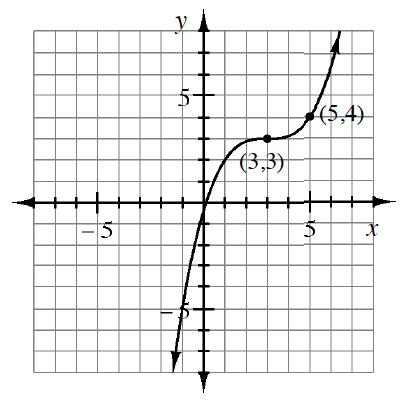### Home > CCA2 > Chapter Ch12 > Lesson 12.1.4 > Problem12-80

12-80.

Write an equation for the curve at right. Then sketch the graph of the inverse of this graph and write its equation.

What parent graph is this similar to?
How is this graph transformed from the parent graph?
Notice that you are given a point other than the locator point so you can solve for $a$.

To find the inverse equation, interchange $x$ and $y$ and solve for $y$.
To graph the inverse equation, choose some points on this graph and interchange $x$ and $y$.